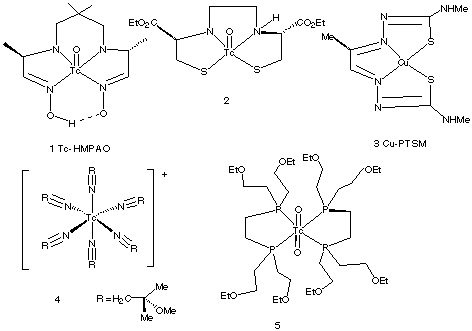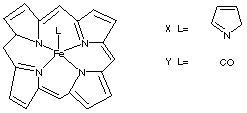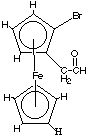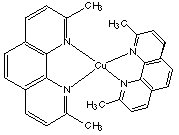1.* (1997 F 4) Certain coordination compounds are used in clinical medicine as heart and brain imaging agents. A selection of these is shown below (from "Chemistry in Britain", October 1994, P. 820).A. Indicate which compounds are chiral. (assume the two oxo groups in (5) are trans.). Write the compound numbers for any chiral compounds you find in Figure 2. B. Compound 2 enters the brain by diffusing through the brain blood barrier; this substance is trapped because it is acted on in the brain by an esterase. A stereoisomer of 2 is not trapped in the brain. Propose a plausible explanation of this fact. C. What is the oxidation state of copper in compound 3? Predict the coordination geometry, and d-electron configuration for 3. D. What is the oxidation state, d-electron configuration, and number of unpaired electrons in compound 4? Click here for solution to problem 1. 2.* (1997 2 2) A. Using any bidentate ("chelating") ligand of your choice, draw a structural formula for a first-row coordination complex which is both chrial and has three unpaired electrons. Also indicate the oxidation state, coordination number, and d electron count of the metal in your complex. Your structure should illustrate the chiral nature of this compound. B. Identify the 3d metal in the following diamagnetic complex anion: M(CN)64-. What is the oxidation state and d-electron count for your metal? Click here for solution to problem 2. 3.* (1997 2 3) Suppose you prepare a complex from propylenediamine, pn (H2NCH(CH3)CH2NH2) and rhodium: Rh(pn)3(ClO4) 3. A. What is the oxidation state of Rh and the d-electron count for this complex? B. Discuss the expected magnetic properties of this compound. Click here for solution to problem 3. 4.* (1996 F 1) What's the maximum number of unpaired electrons any transition metal complex can have? A. Illustrate this for a metal with oxidation state 2+. B. What other condition must be satisfied? Click here for solution to problem 4. 5.* (1996 F 2) Platinum II typically forms diamagnetic, square-planar complexes. Attempts to make octahedral Pt(II) complexes, even with a rigid multicyclic ligand which would force six nitrogens to bind in an octahedral fashion to Pt(II) have not succeeded even though such "sepulchrate" complexes of Co(III) are well known and are very stable. Use ligand field diagrams to explain the reluctance of platinum (II) to form octahedral complexes. Click here for solution to problem 5. 6.* (1996 F 11) A. If you want a transition metal compound to be a good oxidizing agent, should it have a lot of d electrons? Click here for solution to problem 6. 7.* (1996 F 13) Below are structural formulas for two iron porphyrins. The pyridine complex, X, is paramagnetic whereas the carbonyl derivative is diamagnetic. The pyridine complex strongly binds a second pyridine forming a diamagnetic bis-pyridine complex; however, the carbonyl complex, Y, only weakly binds a second carbonyl.A. Offer two reasons for this difference. B. How many unpaired electrons are in the mono pyridine complex? Click here for solution to problem 7. 8.* (1996 2 1) Consider the following compounds; indicate the number of unpaired electrons in each. A. [Pd(NH3)4]2+ C. [Co(CN)5]3- Click here for solution to problem 8. 9.* (1996 2 3) Using only ethylene diamine (en = H2NCH2CH2NH2) and bromide anions as ligands construct a cationic octahedral complex of cobalt (III); your complex cation should have charge +1 and it should be chiral. Draw a three dimensional structure for this coordination complex below on the left. Then draw the structure of a diastereoisomer on the right. Click here for solution to problem 9. 10.* (1995 F 1) E. Give an example of a ligand which has neither p electrons nor non-bonded electron pairs. F. Give an example of a metal complex made only from an anionic aromatic ligand, show the structure, indicate the oxidation state of the metal and the d-electron count. H. Draw a stereochemical structure for a coordination complex which is chiral and has three unpaired electrons. I. Identify the 4d metal in the following diamagnetic complex: M(CO)6. Give its d-electron configuration. Click here for solution to problem 10. 11.* (1995 2 2) A. Draw all possible stereoisomers of [CoCl2(en)(NH3)2]+. Which are chiral? (en = H2NCH2CH2NH2) C. How many unpaired electrons are in the following complexes (assuming all of them have strong-field ligands?): Cu(NH3)42+, CrCl3(THF)3, K3Mn(CN)6. Click here for solution to problem 11. 12.* (1994 F 3C) Name a transition metal cation having 5 unpaired electrons. Click here for solution to problem 12. 13.* (1994 F 7) A novel organometallic anion [Cu(CF3)4]- has recently been prepared. A. What is the shape of this anion? Explain your reasoning. Do you expect this substance to be diamagnetic? B. Calculate the oxidation state, the d electron configuration and the number of unpaired electrons in [N(Et)4]4[V(CN)6] (where Et = C2H5). C. Consider the following ferrocene derivative… What sort of stereoisomers would this substance have? How many stereoisomers would this substance have? How many different 1H NMR signals do you expect? Identify each by marking on the structure.Click here for solution to problem 13. 14.* (1994 2 1) For each of the following complexes: (a) calculate the formal oxidation state of the central metal, (b) give the d electron configuration, (c) deduce the coordination geometry, (d) predict the number of unpaired electrons and (e) give the number and types of steereoisomers, if any (indicate whether these stereoisomers are chiral). I. CoCl42- II. Fe(CN)63- III. Cr(acac)3 IV. Mo(CO)6 Click here for solution to problem 14. 15.* (1994 2 5C) A. Describe how to resolve a racemic mixture of Ru(bipy)32+ into its two enantiomers, and how to make sure that your separation procedure has worked. Click here for solution to problem 15. 16.* (1993 F 1G) Name a complex cation with 3 unpaired electrons, a charge of 3+, and chirality at the metal center. Click here for solution to problem 16. 17.* (1993 F 5) 2,9-dimethyl orthophenanthroline is a reagent used for the selective binding of copper in one of its oxidation states. Which oxidation state of copper does this reagent favor? A copper complex of this ligand is shown below but the charge (and thus oxidation state) is not specified. Discuss this situation in the context of electronic structure and preferred coordination geometry.Click here for solution to problem 17. 18.* (1993 2 1) For each of the following complexes indicate the oxidation state of the central metal, the d electron configuration and the number of unpaired electrons, and whether the structure is chiral or achiral. A. Fe(CN)63- B. Ru(bipy)33+ C. Pt(CN)42- D. p-C6H5Mn(CO)3 E. Ni(PPh3)2(CO)2 F. Cr(acac)3 Click here for solution to problem 18. 19.* (1993 2 3A) Which of the following is rouge and which is colorless? TiO2 and Fe2O3 Click here for solution to problem 19. 20.* (1992 F 25) Consider the diamagnetic complex [M(en)3(ClO4) 3] where M is a 3d metal. A. What is M? B. What is the oxidation state of M? C. How many d electrons? D. Will this complex be a strong oxidizing agent? E. Sketch a picture of all stereoisomers of this complex. F. What features would the 13C NMR spectrum exhibit? Click here for solution to problem 20. 21.* (1992 2 4) Consider two complexes A and B. One is Ni(NH3) 2Br2; the other is Pd(OH2) 2Br2. A is paramagnetic and B is diamagnetic. A. What is the oxidation state of each of the metals? B. How many d electrons does each metal have? C. What is the coordination number of each complex? D. What is the geometry of each complex? E. Which is which? F. Identify the stereoisomers (if any) of each complex. Click here for solution to problem 21. 22.* (1991 2 2) Consider (Cp)M(CO)x. What is M for X=1, X=2, and X=3?   Click here for solution to problem 22. 23.* (1991 2 3) For each of the following complexes, give the oxidation state, number of d electrons, coordination number, number of possible isomers, and state whether the complex is chiral: A. Co(en)33+ B. Pt(NH3)2(Br)2 C. Ni(CO)4 D. Re(CH3)(CO)5 Click here for solution to problem 23. 24.* (1991 2 4) Using any ligand and 3d metal write the formula of a chiral complex with 3 unpaired electrons. Indicate overall charge, oxidation state, and dn. Click here for solution to problem 24. 25.* (1991 2 5) A. Sketch the two enantiomers of Ru(bipy)32+. B. Sketch the bipy ligand. C. How many signals does the proton-decoupled 13C NMR of Ru(bipy)32+ have? D. How many magnetically inequivalent types of H atoms does Ru(bipy)32+ have? E. Is this molecule chiral? Click here for solution to problem 25. 26.* (1990 F 12) Consider a pair of isomeric cationic complexes having the molecular formula [Co(en) 2Br2]ClO 4. One is optically active but the other is not. Write structural formulas showing each isomeric complex but only one of the enantiomers. en = H2NCH2CH2NH2. What is the oxidation state of cobalt in these complexes? How many d electrons are found in each complex? Click here for solution to problem 26.